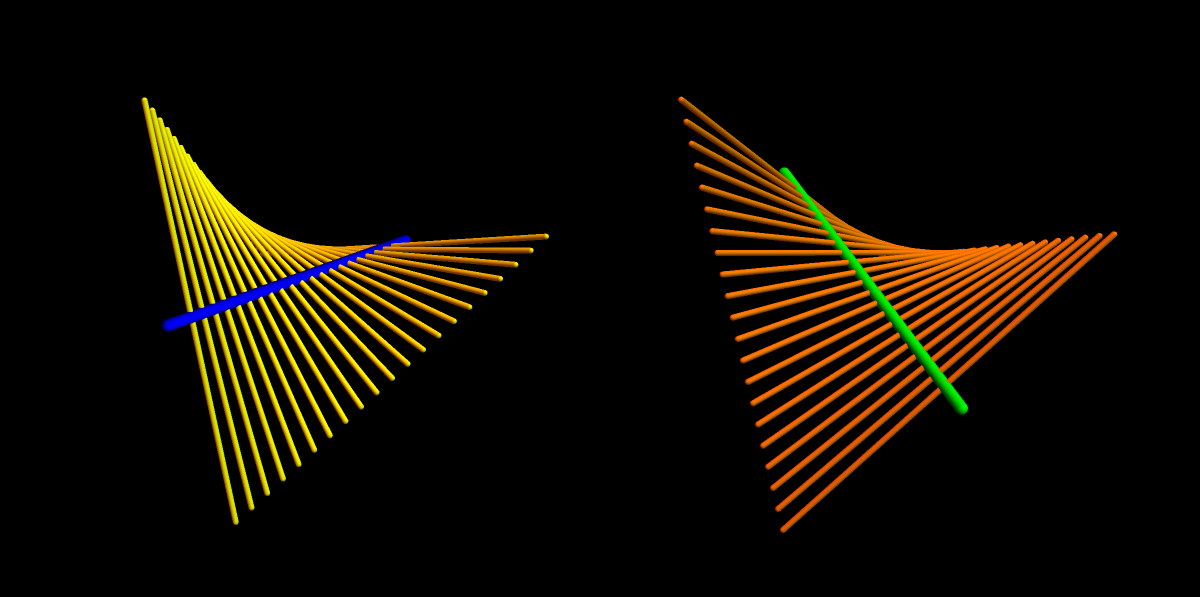A visualization of fxy = fyx . Is there a visual connection (I got this question from Jim Jarreel). The surface obtained by rotating and translating a stick is a saddle. It is symmetric. One can switch x and y and get the same surface. This produces the Claiaut identity.

The usually given proof of Clairaut does not need any visualization in my opinion since it is obvious when doing it in a discrete way. If d_x f(x,y) = [ f(x+h,y)-f(x) ]/h d_y f(x,y) = [ f(x,y+h)-f(x,y) ]/h without taking limits, then d_x d_y = d_y d_x is just algebra. The proof then follows if the limits can be taken.

There is something deeper behind the commutativity: it is a flatness condition. If derivatives are defined on vector fields and space is not flat then covariant derivatives (generalizations of directional derivatives and partial derivatives are directional derivatives) do no more commute. The amount of failure is curvature. Curvature of a surface z=f(x,y) the way agrees with the discriminant D which appears in the second derivative test. This holds if the point is a critical point.